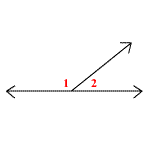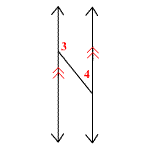# Supplementary Angles

Supplementary angles are two angles whose measures add up to $180°$ .

The two angles of a linear pair , like $\angle 1\text{\hspace{0.17em}}\text{\hspace{0.17em}}\text{and}\text{\hspace{0.17em}}\text{\hspace{0.17em}}\angle 2$ in the figure below, are always supplementary.But, two angles need not be adjacent to be supplementary. In the next figure, $\angle 3\text{\hspace{0.17em}}\text{\hspace{0.17em}}\text{and}\text{\hspace{0.17em}}\text{\hspace{0.17em}}\angle 4$ are supplementary, because their measures add to $180°$ .Example 1:

Two angles are supplementary. If the measure of the angle is twice the measure of the other, find the measure of each angle.

Let the measure of one of the supplementary angles be $a$ .

Measure of the other angle is $2$ times $a$ .

So, measure of the other angle is $2a$ .

If the sum of the measures of two angles is $180°$ , then the angles are supplementary.

So, $a+2a=180°$

Simplify.

$3a=180°$

To isolate $a$ , divide both sides of the equation by $3$ .

$\begin{array}{l}\frac{3a}{3}=\frac{180°}{3}\\ \text{\hspace{0.17em}}\text{\hspace{0.17em}}\text{\hspace{0.17em}}\text{\hspace{0.17em}}a=60°\end{array}$

The measure of the second angle is,

$\begin{array}{l}2a=2×60°\\ \text{\hspace{0.17em}}\text{\hspace{0.17em}}\text{\hspace{0.17em}}\text{\hspace{0.17em}}\text{\hspace{0.17em}}\text{\hspace{0.17em}}\text{\hspace{0.17em}}=120°\end{array}$

So, the measures of the two supplementary angles are $60°$ and $120°$ .

Example 2:

Find $m\angle P\text{\hspace{0.17em}}\text{\hspace{0.17em}}\text{and}\text{\hspace{0.17em}}\text{\hspace{0.17em}}m\angle Q$ if $\angle P\text{\hspace{0.17em}}\text{\hspace{0.17em}}\text{and}\text{\hspace{0.17em}}\text{\hspace{0.17em}}\angle Q$ are supplementary, $m\angle P=2x+15$ , and $m\angle Q=5x-38$ .

The sum of the measures of two supplementary angles is $180°$ .

So, $m\angle P+m\angle Q=180°$

Substitute $2x+15$ for $m\angle P$ and $5x-38$ for $m\angle Q$ .

$2x+15+5x-38=180°$

Combine the like terms. We get:

$7x-23=180°$

Add $23$ to both the sides. We get:

$7x=203°$

Divide both the sides by $7$ .

$\frac{7x}{7}=\frac{203°}{7}$

Simplify.

$x=29°$

To find $m\angle P$ , substitute $29$ for $x$ in $2x+15$ .

$2\left(29\right)+15=58+15$

Simplify.

$58+15=73$

So, $m\angle P=73°$ .

To find $m\angle Q$ , substitute $29$ for $x$ in $5x-38$ .

$5\left(29\right)-38=145-38$

Simplify.

$145-38=107$

So, $m\angle Q=107°$ .